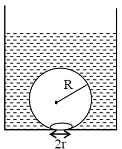Exxamm.com-India’s most trusted exam preparation site for NDA | NEET | NCERT | ENGINEERING | DEFENCE | CBSE | MEDICAL | NTPC | CGL | RRB | SSC | BANKING | NCERT | OLYMPIAD
Q 1720491311.     On heating water, bubbles being formed at the bottom of the vessel detach and rise. Take the bubbles to be spheres of radius R and making a circular contact of radius r with the bottom of the vessel. If r < < R, and the surface tension of water is T, value of r just before bubbles detach is: (density of water is \rho_w)

JEE 2014 Mains Paper - 5AR^2 \sqrt{\frac{\rho_w g}{T}}

BR^2 \sqrt{\frac{3 \rho_w g}{T}}

CR^2 \sqrt{\frac{2\rho_w g}{3 T}}

DR^2 \sqrt{\frac{\rho_w g}{6T}}

0
Success Rate: NAN %